Volumes, Solids of Revolution and Method of Rings/Disks

In trying to find volume of the solid we use same approach as with area problem.

We divide solid into ${n}$ pieces, approximate volume of each piece, take sum of volumes and then take limit as ${n}\to\infty$.

We start from a simple fact: volume of cylinder, with area of base ${A}$ and height ${h}$ is ${V}={A}{h}$.

In particular, circular cylinder, whose base is circle of radius ${r}$ has volume ${V}=\pi{{r}}^{{2}}{h}$. Rectangular box, whose base is rectangle with sides ${a}$ and ${b}$ has volume ${V}={a}{b}{h}$.

Now, consider a general solid ${S}$, that doesn't have a form of cylinder. We first "cut" it into ${n}$ pieces (imagine a cucumber that is not a cylinder, that is cut into ${n}$ pieces). Now, we approximate volume of each piece by volume of cylinder. We estimate the volume of ${S}$ by adding the volumes of the cylinders. We obtain exact volume of ${S}$ though a limiting process in which the number of pieces becomes very large.

We start by intersecting ${S}$ with a plane and obtaining a plane region that is called a cross-section of ${S}$. Let ${A}{\left({x}\right)}$ be the area of the cross-section of ${S}$ in a plane perpendicular to the x-axis and passing through the point ${x}$, where ${a}\le{x}\le{b}$. The cross-sectional area ${A}{\left({x}\right)}$ will vary as ${x}$ increases from a to b.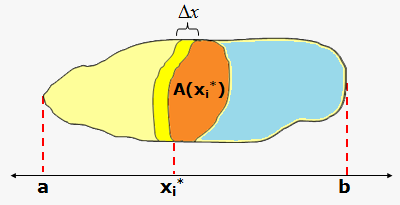Let’s divide ${S}$ into ${n}$ "slabs" of equal width. (Think of slicing a loaf of bread.) If we choose sample points in ${{x}_{{i}}^{{\star}}}$ in ${\left[{x}_{{{i}-{1}}},{x}_{{i}}\right]}$, we can approximate the i-th slab by a cylinder with base area ${A}{\left({{x}_{{i}}^{{\star}}}\right)}$ and "height" $\Delta{x}$.

The volume of this cylinder is ${A}{\left({{x}_{{i}}^{{\star}}}\right)}\Delta{x}$, so an approximation to volume of the i-th slab is ${V}{\left({S}_{{i}}\right)}\approx{A}{\left({{x}_{{i}}^{{\star}}}\right)}\Delta{x}$.

Adding the volumes of these slabs, we get an approximation to the total volume: ${V}\approx{\sum_{{{i}={1}}}^{{n}}}{A}{\left({{x}_{{i}}^{{\star}}}\right)}\Delta{x}$.

This approximation appears to become better and better as ${n}\to\infty$. (Think of the slices as becoming thinner and thinner.) Therefore, we define the volume as the limit of these sums as ${n}\to\infty$: ${V}=\lim_{{{n}\to\infty}}{\sum_{{{i}={1}}}^{{n}}}{A}{\left({{x}_{{i}}^{{\star}}}\right)}\Delta{x}$. But we recognize the limit of Riemann sums as a definite integral and so we have the following definition.

Definition of Volume. Let ${S}$ be a solid that lies between ${x}={a}$ and ${x}={b}$. If the cross-sectional area of ${S}$ through ${x}$ and perpendicular to the x-axis, is ${A}{\left({x}\right)}$, where ${A}$ is a continuous function, then the volume of ${S}$ is ${V}={\int_{{a}}^{{b}}}{A}{\left({x}\right)}{d}{x}$.

It is important to remember that ${A}{\left({x}\right)}$ is the area of a cross-section obtained by slicing through ${x}$ perpendicular to the x-axis.

If we instead slice perpendicular to y-axis then ${V}={\int_{{c}}^{{d}}}{A}{\left({y}\right)}{d}{y}$.

Example 1. Find volume of sphere of radius ${r}$.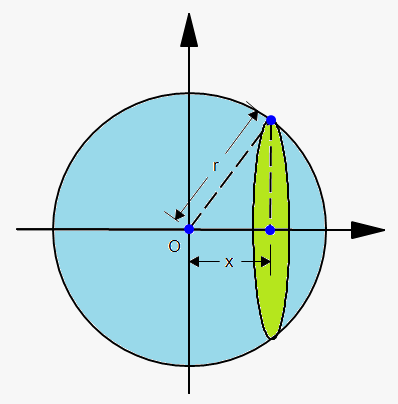If we place the sphere so that its center is at the origin, then the cross-section is circle whose radius (from the Pythagorean
Theorem) is ${y}=\sqrt{{{{r}}^{{2}}-{{x}}^{{2}}}}$.

So, the cross-sectional area is ${A}{\left({x}\right)}=\pi{{y}}^{{2}}=\pi{\left({{r}}^{{2}}-{{x}}^{{2}}\right)}$.

Therefore, ${V}={\int_{{-{r}}}^{{r}}}\pi{\left({{r}}^{{2}}-{{x}}^{{2}}\right)}{d}{x}=\pi{\left({{r}}^{{2}}{x}-\frac{{1}}{{3}}{{x}}^{{3}}\right)}{{\mid}_{{-{r}}}^{{r}}}=$

$=\pi{\left({\left({{r}}^{{2}}\cdot{r}-\frac{{1}}{{3}}\cdot{{r}}^{{3}}\right)}-{\left({{r}}^{{2}}\cdot{\left(-{r}\right)}-\frac{{1}}{{3}}\cdot{{\left(-{r}\right)}}^{{3}}\right)}\right)}=$

$={2}\pi{\left({{r}}^{{3}}-\frac{{1}}{{3}}{{r}}^{{3}}\right)}=\frac{{4}}{{3}}\pi{{r}}^{{3}}$.

Example 2. Find the volume of the solid whose base is a circle of radius ${r}$ and whose cross-sections are equilateral triangles.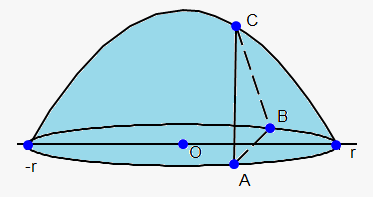Let's take the circle ${{x}}^{{2}}+{{y}}^{{2}}={r}$. Place its center at the origin.

Since B lies on the circle then ${y}=\sqrt{{{{r}}^{{2}}-{{x}}^{{2}}}}$ and so the base of triangle ABC is ${\left|{A}{B}\right|}={2}\sqrt{{{1}-{{x}}^{{2}}}}$. Since triangle is equilateral then its area is ${A}=\frac{{1}}{{2}}\cdot{\sin{{\left({{60}}^{{0}}\right)}}}\cdot{2}\sqrt{{{{r}}^{{2}}-{{x}}^{{2}}}}\cdot{2}\sqrt{{{{r}}^{{2}}-{{x}}^{{2}}}}=$

$=\sqrt{{{3}}}{\left({{r}}^{{2}}-{{x}}^{{2}}\right)}$.

So, cross-sectional area is ${A}{\left({x}\right)}=\frac{{1}}{{2}}\cdot{2}\sqrt{{{1}-{{x}}^{{2}}}}\sqrt{{{3}}}{\left({1}-{{x}}^{{2}}\right)}=\sqrt{{{3}}}{\left({{r}}^{{2}}-{{x}}^{{2}}\right)}$.

Therefore, ${V}={\int_{{-{r}}}^{{r}}}\sqrt{{{3}}}{\left({{r}}^{{2}}-{{x}}^{{2}}\right)}{d}{x}=\sqrt{{{3}}}{\left({{r}}^{{2}}{x}-\frac{{1}}{{3}}{{x}}^{{3}}\right)}{{\mid}_{{-{r}}}^{{r}}}=\frac{{{4}{{r}}^{{3}}}}{{\sqrt{{{3}}}}}$.

Now, let's take a look at solids of revolution.

If we take some function ${y}={f{{\left({x}\right)}}}$ and begin to revolve it around line, then we will obtain solid of revolution. In this case cross-sectional area is area of circle.

Note, that in example 1 we sphere can be treated as solid of revolution: revolving circle ${{x}}^{{2}}+{{y}}^{{2}}={{r}}^{{2}}$ around x-axis.

In general, we calculate the volume of a solid of revolution by using the basic defining formula ${V}={\int_{{a}}^{{b}}}{A}{\left({x}\right)}{d}{x}$ or ${V}={\int_{{c}}^{{d}}}{A}{\left({y}\right)}{d}{y}$ and we find the cross-sectional area in one of the following ways: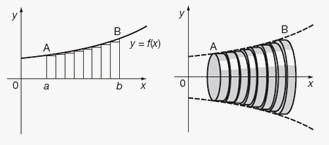1. If the cross-section is a disk then we use method of disks: we find the radius of the disk (in terms of ${x}$ or ${y}$) and use ${A}=\pi{{\left({r}{a}{d}{i}{u}{s}\right)}}^{{2}}$.
2. If the cross-section is a washer (also called ring) then we use method of rings: we find the inner radius ${r}_{\text{in}}$ and outer radius ${r}_{{{o}{u}{t}}}$ from a sketch and compute the area of the washer by subtracting the area of the inner disk from the area of the outer disk: ${A}=\pi{{\left({r}_{{{o}{u}{t}}}\right)}}^{{2}}-\pi{{\left({r}_{{\text{in}}}\right)}}^{{2}}=\pi{\left({{\left({r}_{{{o}{u}{t}}}\right)}}^{{2}}-{{\left({r}_{{\text{in}}}\right)}}^{{2}}\right)}$.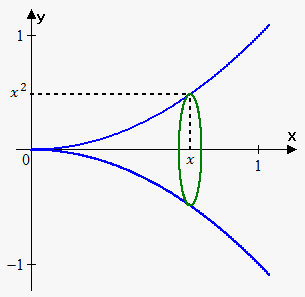Example 3. Find the volume of the solid obtained by rotating about the x-axis the region under the curve ${y}={{x}}^{{2}}$ from 0 to 1.

When we slice through the point ${x}$, we get a disk with radius ${{x}}^{{2}}.$

The area of this cross-section is ${A}{\left({x}\right)}=\pi{{\left({{x}}^{{2}}\right)}}^{{2}}=\pi{{x}}^{{4}}$.

Therefore, ${V}={\int_{{0}}^{{1}}}\pi{{x}}^{{4}}{d}{x}=\frac{\pi}{{5}}{{x}}^{{5}}{{\mid}_{{0}}^{{1}}}=\frac{\pi}{{5}}$.

Example 4. Find the volume of the solid obtained by rotating the region bounded by ${y}={{x}}^{{2}}$, ${y}={x}$ about the x-axis.

First we need to find points of intersection: ${{x}}^{{2}}={x}$. From this we have that ${x}={0}$ and ${x}={1}$. So, points of intersection are ${\left({0},{0}\right)}$ and ${\left({1},{1}\right)}$.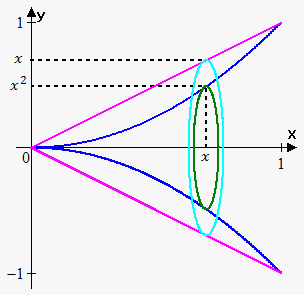Since we rotate around x-axis, then we slice the solid perpendicular to the x-axis.

We have washer (ring) here, so if we slice at ${x}$, then inner radius is ${{x}}^{{2}}$ and outer radius is ${x}$. So, the area of cross-section through ${x}$ is ${A}{\left({x}\right)}=\pi{\left({{\left({x}\right)}}^{{2}}-{{\left({{x}}^{{2}}\right)}}^{{2}}\right)}=\pi{\left({{x}}^{{2}}-{{x}}^{{4}}\right)}$.

Bounds of integration are 0 and 1 (we integrate with respect to ${x}$).

Therefore, ${V}={\int_{{0}}^{{1}}}\pi{\left({{x}}^{{2}}-{{x}}^{{4}}\right)}{d}{y}=\pi{\left(\frac{{1}}{{3}}{{x}}^{{3}}-\frac{{1}}{{5}}{{x}}^{{5}}\right)}{{\mid}_{{0}}^{{1}}}=\pi{\left(\frac{{1}}{{3}}-\frac{{1}}{{5}}\right)}=\frac{{2}}{{15}}\pi$.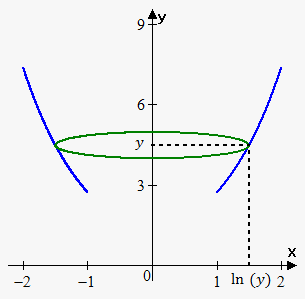Example 5. Find the volume of the solid obtained by rotating about the y-axis the region under the curve ${y}={{e}}^{{x}}$ from 1 to 2.

Since we rotate around y-axis, we need function in terms of ${y}$ because cross-sectional area depends on ${y}$: ${x}={\ln{{\left({y}\right)}}}$.

When we slice through the point ${y}$, we get a disk with radius ${{\left({\ln{{\left({y}\right)}}}\right)}}^{{2}}$.

The area of this cross-section is ${A}{\left({y}\right)}=\pi{{\left({\ln{{\left({y}\right)}}}\right)}}^{{2}}=\pi{{x}}^{{4}}$.

Bounds of integration are from ${{e}}^{{1}}$ to ${{e}}^{{2}}$.

Therefore, ${V}={\int_{{e}}^{{{{e}}^{{2}}}}}\pi{{\left({\ln{{\left({y}\right)}}}\right)}}^{{2}}{d}{y}$.

This integral can be easily evaluated by applying integration by parts twice:

${V}=\pi{e}{\left({2}{e}-{1}\right)}$.

Example 6. Find the volume of the solid obtained by rotating the region bounded by ${y}=\sqrt{{{x}}}$, ${y}=\frac{{x}}{{2}}$ about the y-axis.

First we need to find points of intersection: $\sqrt{{{x}}}=\frac{{x}}{{2}}$ or ${x}=\frac{{{{x}}^{{2}}}}{{4}}$. From this we have that ${x}={0}$ and ${x}={4}$. So, points of intersection are ${\left({0},{0}\right)}$ and ${\left({4},{2}\right)}$.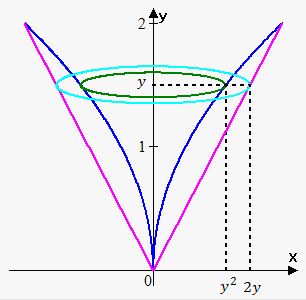Since we rotate around y-axis, then we slice the solid perpendicular to the y-axis and rotate with respect to ${y}$.

In this case cross-sectional area is function of ${y}$ and we need to express functions in terms of ${y}$: ${x}={{y}}^{{2}}$ and ${x}={2}{y}$.

We have washer (ring) here, so if we slice at height ${y}$, then inner radius is ${{y}}^{{2}}$ and outer radius is ${2}{y}$. So, the area of cross-section through ${y}$ is ${A}{\left({y}\right)}=\pi{\left({{\left({2}{y}\right)}}^{{2}}-{{\left({{y}}^{{2}}\right)}}^{{2}}\right)}=\pi{\left({4}{{y}}^{{2}}-{{y}}^{{4}}\right)}$.

Bounds of integration are 0 and 2 (we integrate with respect to ${y}$).

Therefore, ${V}={\int_{{0}}^{{2}}}\pi{\left({4}{{y}}^{{2}}-{{y}}^{{4}}\right)}{d}{y}=\pi{\left(\frac{{4}}{{3}}{{y}}^{{3}}-\frac{{1}}{{5}}{{y}}^{{5}}\right)}{{\mid}_{{0}}^{{2}}}=\pi{\left(\frac{{32}}{{3}}-\frac{{32}}{{5}}\right)}=\frac{{64}}{{15}}\pi$.

Example 7. Find the volume of the solid obtained by rotating the region bounded by ${y}={{x}}^{{2}}$, ${y}={x}$ about the ${y}={2}$.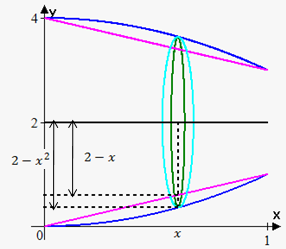As in example 4 points of intersection are ${\left({0},{0}\right)}$ and ${\left({1},{1}\right)}$, cross-section is a washer, but this time the inner radius is ${2}-{x}$ and outer radius is ${2}-{{x}}^{{2}}$.

The cross-sectional area is ${A}{\left({x}\right)}=\pi{{\left({2}-{{x}}^{{2}}\right)}}^{{2}}-\pi{{\left({2}-{x}\right)}}^{{2}}=\pi{\left({{x}}^{{4}}-{5}{{x}}^{{2}}+{4}{x}\right)}$.

Therefore, ${V}={\int_{{0}}^{{1}}}\pi{\left({{x}}^{{4}}-{5}{{x}}^{{2}}+{4}{x}\right)}{d}{x}=\pi{\left(\frac{{1}}{{5}}{{x}}^{{5}}-\frac{{5}}{{3}}{{x}}^{{3}}+{2}{{x}}^{{2}}\right)}{{\mid}_{{0}}^{{1}}}=$

$=\pi{\left(\frac{{1}}{{5}}-\frac{{5}}{{3}}+{2}\right)}=\frac{{{8}\pi}}{{15}}$.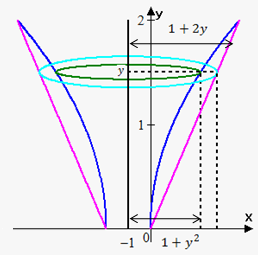Example 8. Find the volume of the solid obtained by rotating the region bounded by the curves ${y}=\sqrt{{{x}}}$ and ${y}=\frac{{x}}{{2}}$ about the line ${x}=-{1}$.

As in Example 6 points of intersection are ${\left({0},{0}\right)}$ and ${\left({4},{2}\right)}$.

Since we rotate about vertical line, then we need to express functions in terms of ${y}$: ${x}={{y}}^{{2}}$ and ${x}={2}{y}$.

Now let's see what radii are. The distance from y-axis to inner function is ${{y}}^{{2}}$, this means that distance from line ${x}=-{1}$ to inner function is ${1}+{{y}}^{{2}}$.

Similarly the distance from line ${x}=-{1}$ to outer function (outer radius) is ${1}+{2}{y}$.

Note, that it is very important to draw a sketch to correctly determine radii.

So, cross-sectional area is ${A}{\left({y}\right)}=\pi{\left({{\left({1}+{2}{y}\right)}}^{{2}}-{{\left({1}+{{y}}^{{2}}\right)}}^{{2}}\right)}=\pi{\left({4}{y}+{2}{{y}}^{{2}}-{{y}}^{{4}}\right)}$.

Therefore, ${V}={\int_{{0}}^{{2}}}\pi{\left({4}{y}+{2}{{y}}^{{2}}-{{y}}^{{4}}\right)}{d}{y}=\pi{\left({2}{{y}}^{{2}}+\frac{{2}}{{3}}{{y}}^{{3}}-\frac{{1}}{{5}}{{y}}^{{5}}\right)}{{\mid}_{{0}}^{{2}}}=\pi{\left({8}+\frac{{16}}{{3}}-\frac{{32}}{{5}}\right)}=\frac{{{104}\pi}}{{15}}$.

Example 9. Find volume of a cap of a sphere with radius ${r}$ and height ${h}$.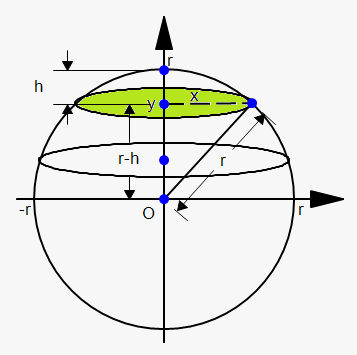We need to find the top portion of the sphere of height ${h}$, so, the cap starts at ${y}={r}-{h}$ and ends at ${y}={r}$.

If we slice perpendicular to y axis then each cross-section is circle with radius (according to Pythagorean theorem) ${{x}}^{{2}}={{r}}^{{2}}-{{y}}^{{2}}$. Therefore, cross-sectional area is ${A}{\left({y}\right)}=\pi{{x}}^{{2}}=\pi{\left({{r}}^{{2}}-{{y}}^{{2}}\right)}$.

Thus, ${V}={\int_{{{r}-{h}}}^{{r}}}\pi{\left({{r}}^{{2}}-{{y}}^{{2}}\right)}{d}{y}=\pi{\left({{r}}^{{2}}{y}-\frac{{1}}{{3}}{{y}}^{{3}}\right)}{{\mid}_{{{r}-{h}}}^{{r}}}=$

$=\pi{\left({\left({{r}}^{{2}}\cdot{r}-\frac{{1}}{{3}}\cdot{{r}}^{{3}}\right)}-{\left({{r}}^{{2}}\cdot{\left({r}-{h}\right)}-\frac{{1}}{{3}}\cdot{{\left({r}-{h}\right)}}^{{3}}\right)}\right)}=$

$=\pi{{h}}^{{2}}{\left({r}-\frac{{1}}{{3}}{h}\right)}$. Note that when ${h}={2}{r}$, we obtain volume of all sphere $\frac{{4}}{{3}}\pi{{r}}^{{3}}$.